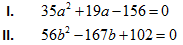New Students Offer - Use Code HELLO

# Quadratic Equation Questions with Explanations### Question 1.

In the following question two equations (A) and (B) given. You have to solve both:A. If a > b
B. If a < b
C. If a = b
D. If a ≥ b
E. If a ≤ b
Ans. C.
Solution:

### Question 2.

In the following question two equations (A) and (B) given. You have to solve both:
A. If a > b
B. If a < b
C. If a = b
D. If a ≥ b
E. If a ≤ b
Ans. E.
Solution:

### Question 3.

In the following question two equations (A) and (B) given. You have to solve both:
A. If a > b
B. If a < b
C. If a = b
D. If a ≥ b
E. If a ≤ b
Ans. E.
Solution:

### Question 4.

In the following question two equations (A) and (B) given. You have to solve both:
A. If a > b
B. If a < b
C. If a = b
D. If a ≥ b
E. If a ≤ b
Ans. D.
Solution:

### Question 5.

In the following question two equations (A) and (B) given. You have to solve both:

A. If a > b
B. If a < b
C. If a = b
D. If a ≥ b
E. If a ≤ b
Ans. C.
Solution:

### Question 6.

In the following question two equations (A) and (B) given. You have to solve both:
A. If a > b
B. If a < b
C. If a = b
D. If a ≥ b
E. If a ≤ b
Ans. B.
Solution:

### Question 7.

In the following question two equations (A) and (B) given. You have to solve both:
A. If a > b
B. If a < b
C. If a = b
D. If a ≥ b
E. If a ≤ b
Ans.  B.
Solution:

### Question 8.

In the following question two equations (A) and (B) given. You have to solve both:
A. If a > b
B. If a < b
C. If a = b
D. If a ≥ b
E. If a ≤ b
Ans. C.
Solution:

### Question 9.

In the following question two equations (A) and (B) given. You have to solve both:
A. If a > b
B. If a < b
C. If a = b
D. If a ≥ b
E. If a ≤ b
Ans. E.
Solution:

### Question 10.

In the following question two equations (A) and (B) given. You have to solve both:
A. If a > b
B. If a < b
C. If a = b
D. If a ≥ b
E. If a ≤ b
Ans. C.
Solution: